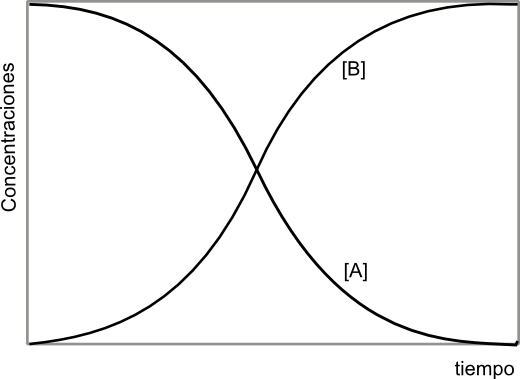A very simple example of autocatalysis can be found in the following reaction: \begin{equation} A+B\stackrel{k}{\rightarrow}2B \;\; \Rightarrow r=-\frac{dA}{dt}=k[A][B] \end{equation} In the initial instants there is an increase in the concentration of B, which causes a rapid increase in velocity (note that B is reactant and product). However, as the reaction progresses, reagent A disappears and the speed begins to decrease, until it becomes zero, when this component disappears. The evolution of the concentrations of A and B over time is a sigmoid curve, which responds to the following equation: \begin{equation} kt=\frac{1}{[A]_0+[B]_0}ln\frac {[B]/[B]_0}{[A]/[A]_0} \end{equation}